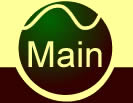#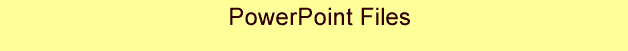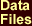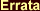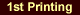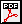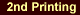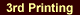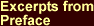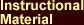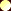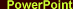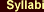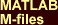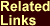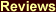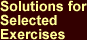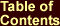Further Information James C. Spall Johns Hopkins University Applied Physics Laboratory 11100 Johns Hopkins Rd. Laurel, MD 20723-6099 USA

The PowerPoint slides here have been used as an aid in teaching from the book Introduction to Stochastic Search and Optimization. These slides are a complement to the book and are not intended to be a stand-alone treatment of the material in the text. The coverage of the chapters is not even; the slides for some chapters are more thoroughly developed than for other chapters. Development and modification of these slides is an ongoing process. Many fundamental topics in the book are not covered in these slides. Nonetheless, students and instructors are free to use these slides as appropriate. This use is restricted to noncommercial applications for self-study or for courses at educational institutions.

© 2012 The Johns Hopkins University
Applied Physics Laboratory (JHU/APL)
 CHAPTERS 1 – 17Chapter 1. Stochastic Search and Optimization: Motivation and Supporting ResultsChapter 2.Chapter 3. Recursive Estimation for Linear ModelsChapter 4. Stochastic Approximation for Nonlinear Root-FindingChapter 5. Stochastic Gradient Form of Stochastic ApproximationChapter 6. Stochastic Approximation and the Finite-Difference MethodChapter 7. Simultaneous Perturbation Stochastic ApproximationChapter 8. Annealing-Type AlgorithmsChapter 9. Evolutionary Computation I: Genetic AlgorithmsChapter 10. Evolutionary Computation II: General Methods and TheoryChapter 11. Reinforcement Learning via Temporal DifferencesChapter 12. Statistical Methods for Optimization in Discrete ProblemsChapter 13. Model Selection and Statistical InformationChapter 14. Simulation-Based Optimization I: Regeneration, Common Random Numbers, and Selection MethodsChapter 15. Simulation-Based Optimization II: Stochastic Gradient and Sample Path MethodsChapter 16. Markov Chain Monte CarloChapter 17. Optimal Design for Experimental Inputs APPENDICES A – EAppendix A. Selected Results from Multivariate AnalysisAppendix B. Some Basic Tests in StatisticsAppendix C. Probability Theory and ConvergenceAppendix D. Random Number GenerationAppendix E. Markov Processes SUPPLEMENTAL SLIDES FOR COURSE IN SIMULATION AND MONTE CARLO METHODSSimulation and Monte Carlo: Some Introductory Remarks